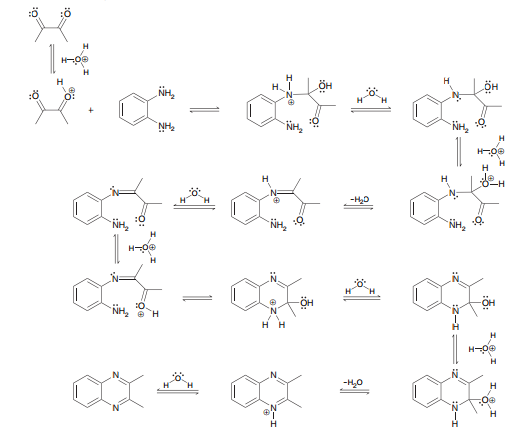Problem: Draw curved arrows for each step of the following mechanism:

FREE Expert Solution
96% (425 ratings)
Problem Details

Draw curved arrows for each step of the following mechanism:What scientific concept do you need to know in order to solve this problem?

Our tutors have indicated that to solve this problem you will need to apply the Reaction Mechanism concept. You can view video lessons to learn Reaction Mechanism Or if you need more Reaction Mechanism practice, you can also practice Reaction Mechanism practice problems .

What is the difficulty of this problem?

Our tutors rated the difficulty of Draw curved arrows for each step of the following mechanism:... as high difficulty.

How long does this problem take to solve?

Our expert Organic tutor, Chris took 6 minutes to solve this problem. You can follow their steps in the video explanation above.

What professor is this problem relevant for?

Based on our data, we think this problem is relevant for Professor Chen's class at CSU.

What textbook is this problem found in?

Our data indicates that this problem or a close variation was asked in . You can also practice practice problems .